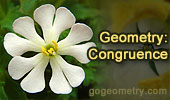# Online Geometry: Congruence, Rotation, Translation, Reflection - Page 6Proposed Problem 78: Angles of a Circle. Perpendicular and parallel lines, Midpoint, Diameter, Chord, Cyclic quadrilateral, Congruence.

Interactive Hinged tessellation. Square tessellation - Dynamic Geometry.
Please be patient while the applet loads on your computer. This Java applet requires Java 1.3 or higher.

Archimedes' Book of Lemmas.
Proposition 5,
Arbelos, Archimedean Twins.

I have used Geometry Expressions, the world's first Interactive Symbolic Geometry System, to visualize the Archimedean Twins and check out a variety of conjectures.

Proposed Problem 60: Isosceles triangle and angles.

Proposed Problem 49. Angles and right triangle.

Proposed Problem 48. Angles and triangles.

Proposed Problem 47. Angles and triangles.

Proposed Problem 46. Angles and triangles.

Proposed Problem 45. Angles and triangles.

Proposed Problem 44. Angles and triangles.

Proposed Problem 43. Angles and triangles.

Proposed Problem 42. Angles and triangles.

Proposed Problem 37.
Right Triangle, altitude, incircles, incenters, and orthocenter.

Previous | 1 | 2 | 3 | 4 | 5 | 6 | 7 | 8 | 9 | 10 | Next

 Home | Geometry | Problems | Email | By Antonio Gutierrez